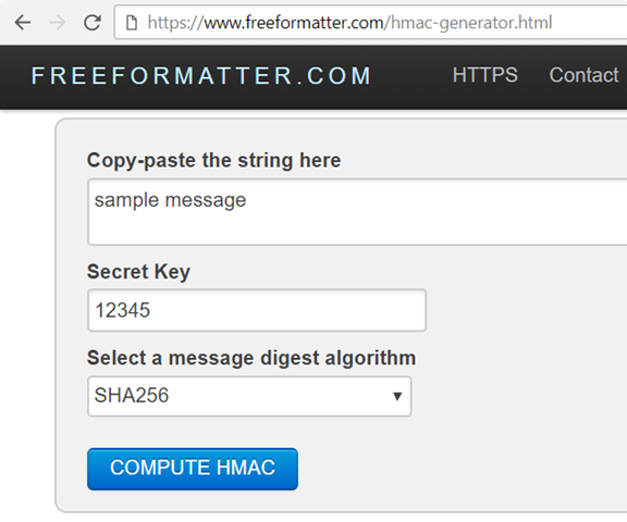# HMAC and Key Derivation Functions (KDF)

Simply calculating hash_func(key + msg) to obtain a MAC (message authentication code) is considered insecure (see the details). It is recommended to use the HMAC algorithm instead, e.g. HMAC-SHA256 or HMAC-SHA3-512 or other secure MAC algorithm.

## What is HMAC?

HMAC = Hash-based Message Authentication Code (MAC code, calculated using a cryptographic hash function):

HMAC(key, msg, hash_func) -> hash


The results MAC code is a message hash mixed with a secret key. It has the cryptographic properties of hashes: irreversible, collision resistant, etc.

The hash_func can be any cryptographic hash function like SHA-256, SHA-512, RIPEMD-160, SHA3-256 or BLAKE2s.

HMAC is used for message authenticity, message integrity and sometimes for key derivation.

## Key Derivation Functions (KDF)

Key derivation function (KDF) is a function which transforms a variable-length password to fixed-length key (sequence of bits):

function(password) -> key


As very simple KDF function, we can use SHA256: just hash the password. Don't do this, because it is insecure. Simple hashes are vulnerable to dictionary attacks.

As more complicated KDF function, you can derive a password by calculating HMAC(salt, msg, SHA256) using some random value called "salt", which is stored along with the derived key and used later to derive the same key again from the password.

Using HKDF (HMAC-based key derivation) for key derivation is less secure than modern KDFs, so experts recommend using stronger key derivation functions like PBKDF2, Bcrypt, Scrypt and Argon2. We shall discuss all these KDF functions later.

## HMAC Calculation - Example

To get a better idea of HMAC and how it is calculated, try this online tool: https://www.freeformatter.com/hmac-generator.htmlPlay with calculating HMAC('sample message', '12345', 'SHA256'):

HMAC('sample message', '12345', 'SHA256') =